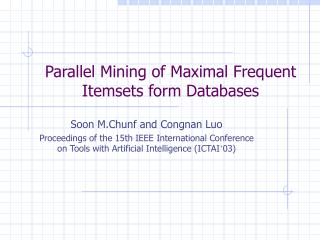DownloadDownload PresentationParallel Mining of Maximal Frequent Itemsets form Databases

# Parallel Mining of Maximal Frequent Itemsets form Databases

Télécharger la présentation## Parallel Mining of Maximal Frequent Itemsets form Databases

- - - - - - - - - - - - - - - - - - - - - - - - - - - E N D - - - - - - - - - - - - - - - - - - - - - - - - - - -
##### Presentation Transcript

1. Parallel Mining of Maximal Frequent Itemsets form Databases Soon M.Chunf and Congnan Luo Proceedings of the 15th IEEE International Conference on Tools with Artificial Intelligence (ICTAI’03)

2. Outline • Introduction • Max-Miner Algorithm • Parallel Max-Miner (PMM) Algorithm • Performance Evaluation • Conclusion

3. Introduction (1) • In mining association rules, the most time-consuming job is finding all frequent itemsets from a large database with respect to a given minimum support • In Apriori, the subset-infrequency based pruning step prevents many candidate k-itemsets from being counted in each pass k • In Apriori-like algorithms, if there is a frequent itemset with length l, then they will generate and count its 2l subsets.

4. Introduction (2) • Our basic idea is that if we find a large frequent itemset early, we can avoid counting all its subsets because they are all frequent • We propose a parallel algorithm, named Parallel Max-Miner (PMM), for mining maximal frequent items • The PMM requires multiple passes over the database, like the Count Distribution algorithm, need synchronization between nodes at every pass end

5. Max-Miner algorithm • Unlike Apriori, the Max-Miner algorithm extracts only the maximal frequent itemset • Superset-frequency based pruning • Max-miner always attempts to look ahead in order to identify large frequent itemsets early • So all subsets of these discovered frequent itemsets can be pruned form the search space

6. Set-enumeration tree of Max-Miner (1)

7. Set-enumeration tree of Max-Miner (2) • Each node in the tree is called a candidate group • A candidate group g consists of two components which are actually two itemsets • The first itemset is called the head of the group and denoted by h(g) • The second itemset is called the tail of the group and denoted by t(g) • t(g) is an ordered set and contains all the items not in h(g) but can potentially appear in any subnode derived from node g

8. The main procedure of Max-Miner (1) • From the root of the tree at level 0, count the support of 1-itemsets. • Only the 1-itemsets which are frequent can be enumerated at level 1 • 4 nodes are generated at level 1 if 1, 2, 3, and 4 are all frequent 1-itemsets • For the node g1, we need to count the support of {h(g1) t(g1)}={1,2,3,4} • If the support of {h(g1) t(g1)} is equal or greater than minsup, then we do not need to expand the tree from the node g1 anymore

9. The main procedure of Max-Miner (2) • At any node g, if {h(g) t(g)} is not frequent, for each item I in t(g), we check if {h(g) i} is frequent • If {h(g) i} is frequent, a corresponding subnode is generated • We notice that for a candidate group node g, if an item appears last in the tail of g in ordering, it will appear in most offsprings of the node g • To discover the maximal frequent itemsets early, we better order the subnodes of each node in ascending order of their support

10. Parallel Max-Miner (PMM) algorithm • The database is evenly divided into N partitions {D0, D1, D2, …, DN-1}, one for each of the N nodes {P0, P1, P2, …, PN-1} • Each node has the same number of transactions allocated • PMM requires multiple passes over database • For each pass k, all the nodes have exactly the same set of candidate groups, Ck. • Each node count the support of Ck in local database, independently • At the end of each pass, all nodes exchange the count information so that they can generate the same set of Ck-1 for the next pass

11. Performance Evaluation Speedup of PMM Sizeup of PMM

12. Conclusion • We proposed a parallel maximal frequent itemset mining algorithm, Parallel Max-Miner, for shared-nothing multiprocessor systems • Drawback: quire synchronization between nodes to exchange the count information at the end of every pass